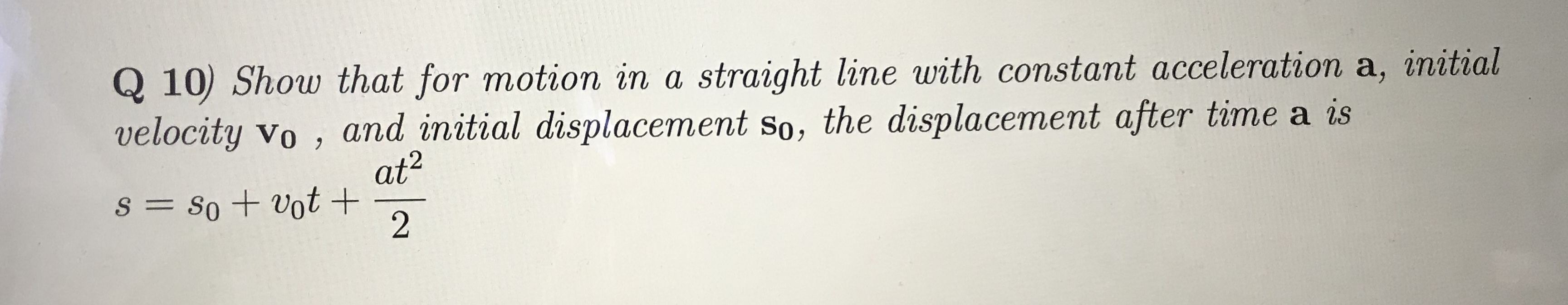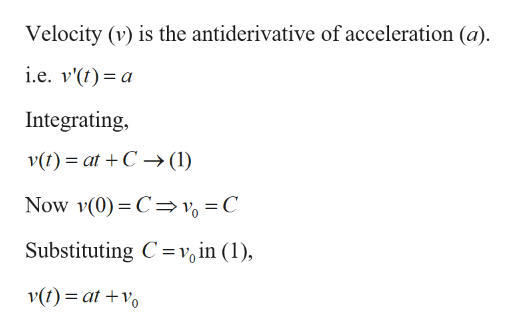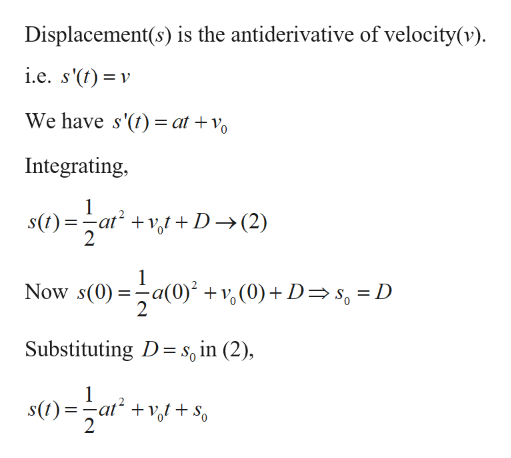# Q 10 Show that for motion in a straight line with constant acceleration a, initialvelocity vo , and initial displacement so, the displacement after time a isat2S= So + vot+2

Question
44 viewshelp_outlineImage TranscriptioncloseQ 10 Show that for motion in a straight line with constant acceleration a, initial velocity vo , and initial displacement so, the displacement after time a is at2 S= So + vot+ 2 fullscreen
check_circle

Step 1
Step 2help_outlineImage TranscriptioncloseVelocity (v) is the antiderivative of acceleration (a) i.e. v'(t a Integrating, v(t)atC (1) Now v(0) C v = C Substituting C v, in (1) v(t) at +o fullscreen
Step 3help_outlineImage TranscriptioncloseDisplacement(s) is the antiderivative of velocity(v) i.e. s'(t) v We have s'() at + Integrating, votD-(2) Now s(0)a( (0)D s = D Substituting D \$6 in (2), s(t)at + S fullscreen

### Want to see the full answer?

See Solution

#### Want to see this answer and more?

Solutions are written by subject experts who are available 24/7. Questions are typically answered within 1 hour.*

See Solution
*Response times may vary by subject and question.
Tagged in